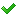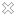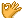•闪存
•博客
• 发言小组
• 投递新闻
• 提问博问
• 添加收藏
•文库

3120很奇怪 去掉 print(list(g)) 之后 print(i) 才能执行 否则就不执行

def y(list): for i in list: yield i def exec(g): print(list(g)) for i in g: print(i) t = (123, 'xyz'
0120很奇怪 去掉 print(list(g)) 之后 print(i) 才能执行 否则就不执行

def y(list): for i in list: yield i def exec(g): print(list(g)) for i in g: print(i) t = (123, 'xyz'
2KOTLIN新手入门 关于内部类的属性set get方法

package practice open class Parent{ open val name="BABA" val money=5 get(){ println("获取B属性值") return
215JS框架第一句的解析 求解释

var toString={}.toString,slice=[].slice,UA=_W_.navigator.userAgent.toLowerCase(); 求解析
4昨天在慕客网做javascript练习遇到的问题 关于remove(this)的疑问

<a href="javascript:;" onclick="remove(this);"></a> 这样的一句 在microsoft edge以及 safari浏览器中 点击a标签会将a标签自己删